Irrational Numbers

Chapter 1 Class 9 Number Systems
Concept wise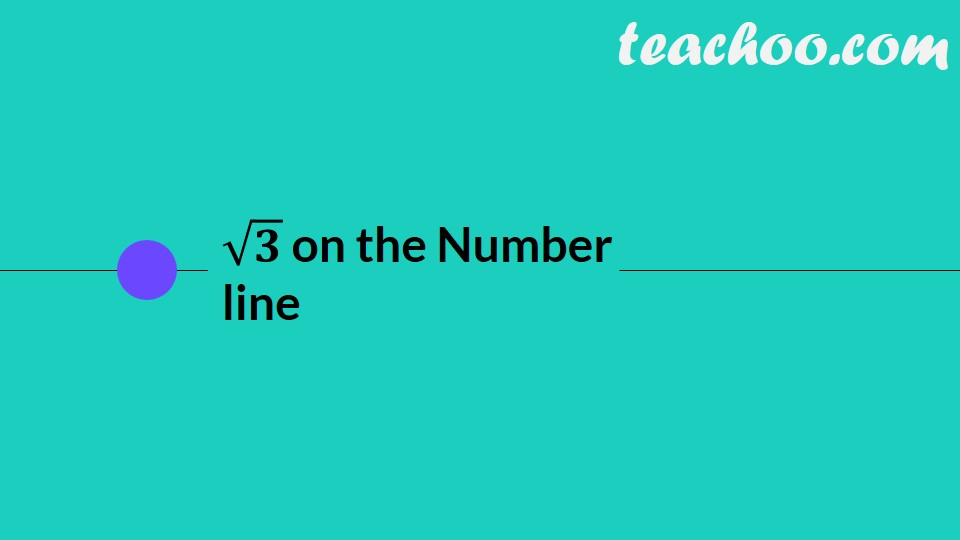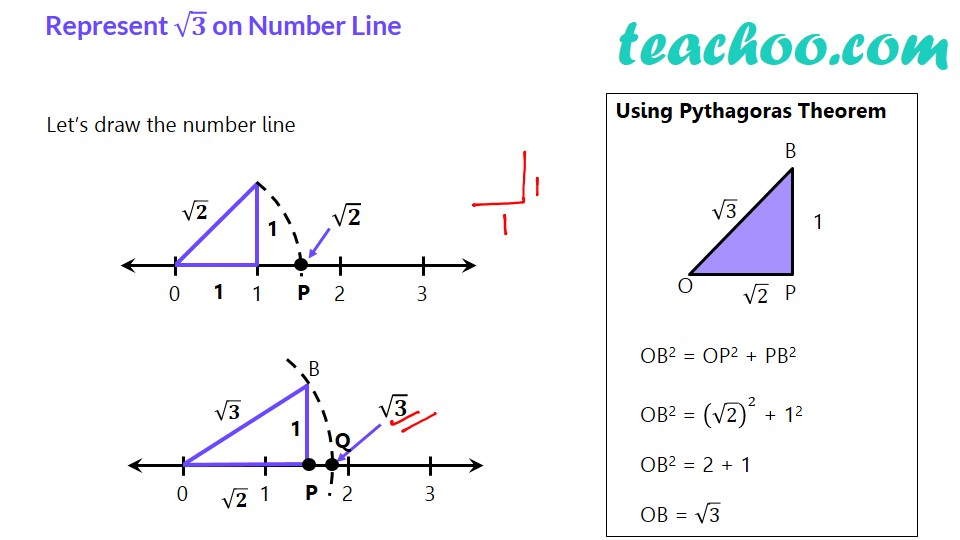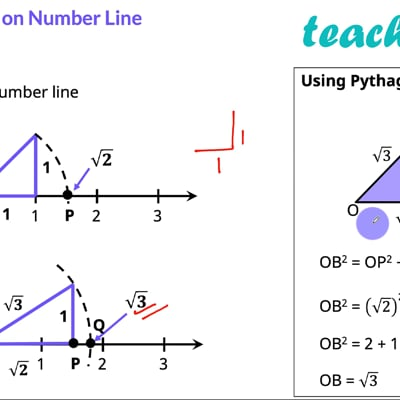This video is only available for Teachoo black users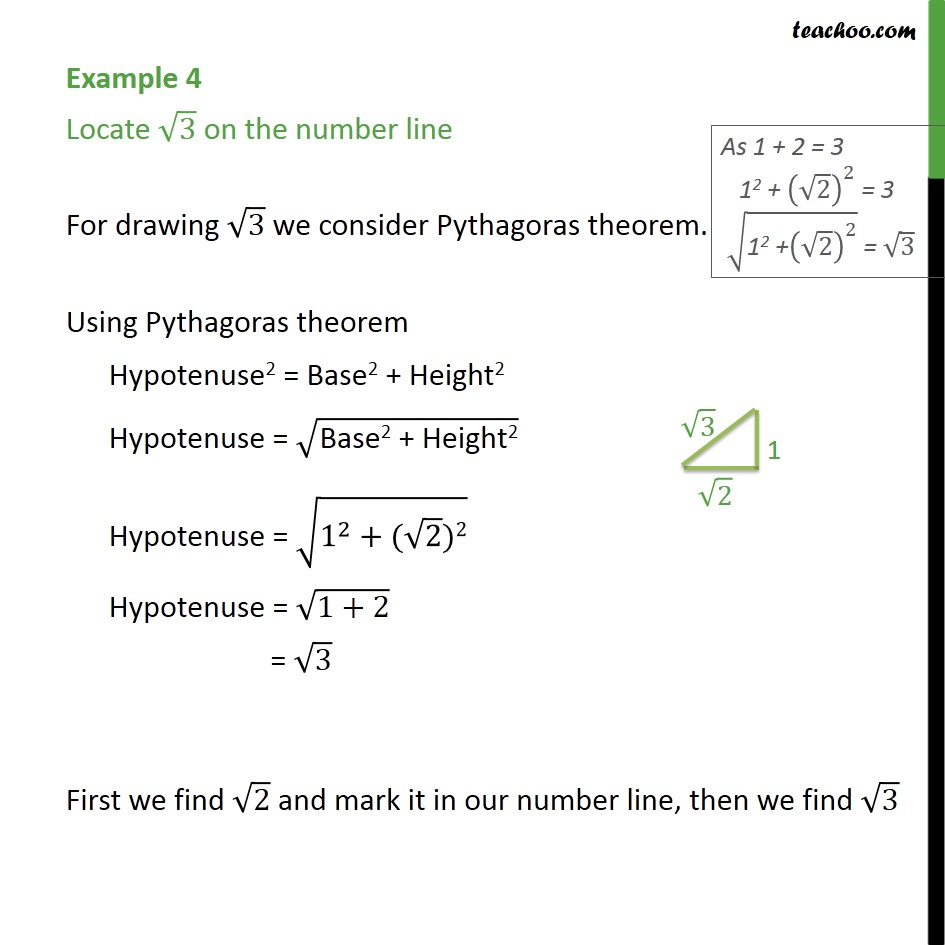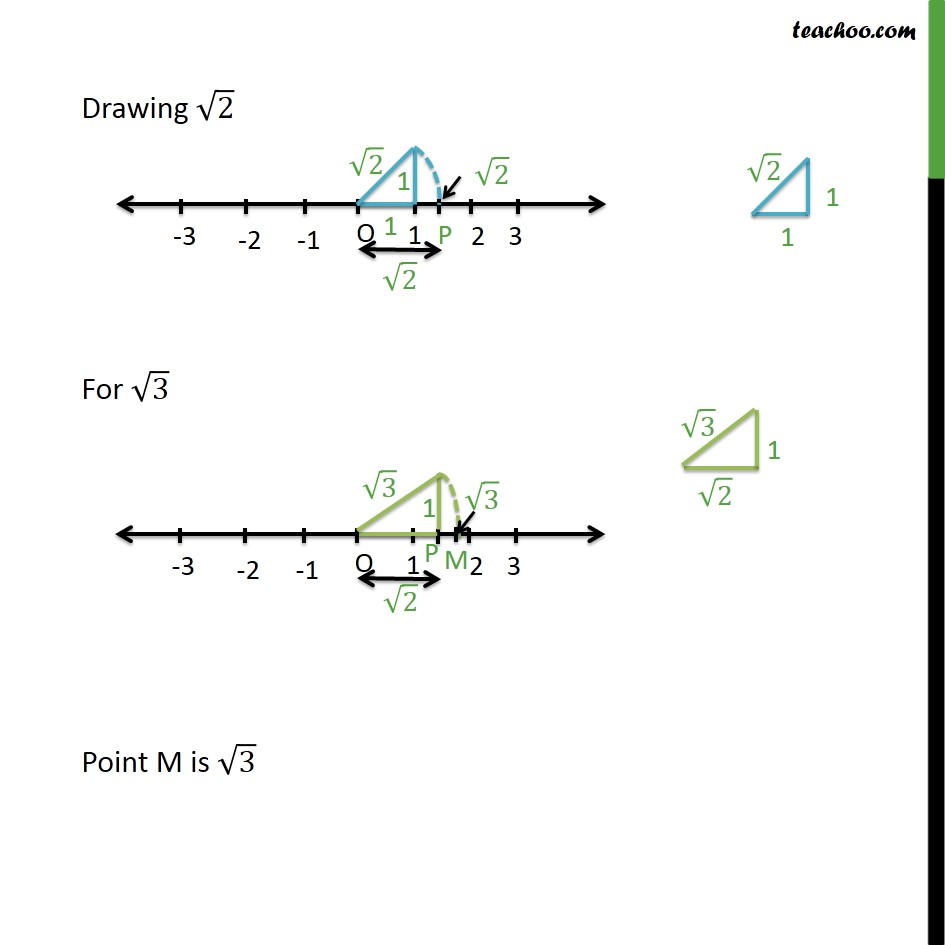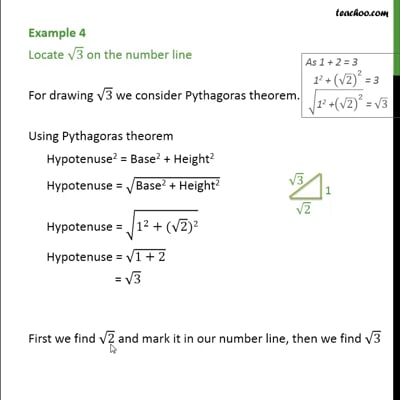This video is only available for Teachoo black users

Introducing your new favourite teacher - Teachoo Black, at only ₹83 per month

### Transcript

√𝟑 on the Number line Represent √𝟑 on Number Line Let’s draw the number line Using Pythagoras Theorem OB2 = OP2 + PB2 OB2 = (√2)^2 + 12 OB2 = 2 + 1 OB = √3 Example 4 Locate 3 on the number line For drawing 3 we consider Pythagoras theorem. Using Pythagoras theorem Hypotenuse2 = Base2 + Height2 Hypotenuse = ("Base2 + Height2" ) Hypotenuse = (1^2+( 2)2) Hypotenuse = (1+2) First we find 2 and mark it in our number line, then we find 3 Drawing 2 For 3 Point M is 3